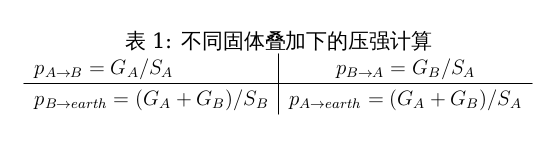# 教案：一道有关叠加固体的压强的比问题

## 引入

（提问)

( $p=\frac{F}{S}$ )（提问)（提问)

（$\frac{p_A}{p_B}$ = $G_A / G_B \cdot S_B / A_B$）（提问)对于下面这幅图我们需要求的压强有几个？

（4个）

( $p_{A \Rightarrow B} , p_{B \Rightarrow earth} , p_{B \Rightarrow A} , p_{A \Rightarrow earth}$ )

## 主体

$总结$ 让我们来总结一下我们这道题目。大家再次回味一下我们的解题过程，其实我们就是抓住了两组量之间的关系来进行比较的。

## 拓展

(视时间多少决定是否讲)

(每个金属片表面所受的压强之比是$1:1$,桌面所受的压强为$2G/S$)

Written on October 2, 2012